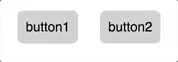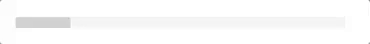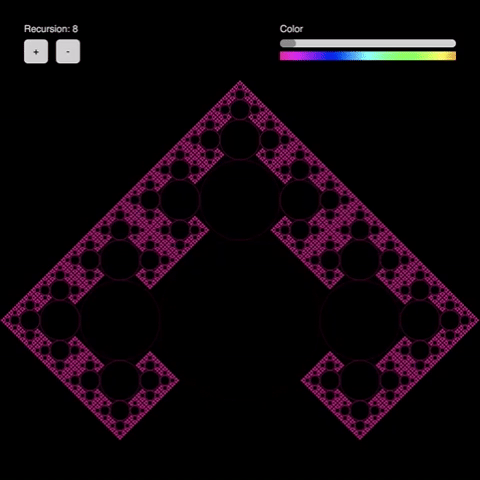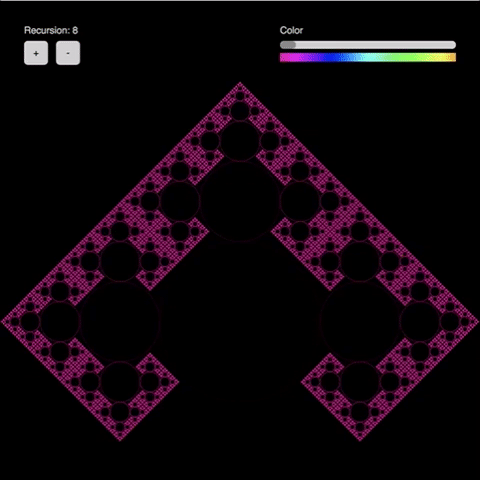# ICM Week3

The word ‘algorithmic design’ in the requirement of this week’s assignment reminded me of ‘Fractals’, which exhibit similar patterns at increasingly small scale, according to the Wikipedia: https://en.wikipedia.org/wiki/Fractal So I planned to create a fractal controllable by UI elements.

I first developed the visual design part and the interface part separately and combined them later.

Here is the process of the project.

ALGORITHMIC DESIGN: A FRACTAL

Before beginning the coding I googled some fractal images for the reference. Following pictures are the examples.

Inspired by the Mandelbrot set, I came up with the idea of a fractal that consists of circles. Actually, it was a simple version of Mandelbrot set, in the pattern of which, a circle has three half-sized circles on its edges in 90˚, 180˚, and 270˚ directions.

First, I drew just a two-level fractal in a manual way to figure out the mathematical rule.

``` function draw() { background(0); noFill(); translate(width/2, (height/2)+rad/2); ellipse(0,0,r,r); // draw the biggest circle push(); translate(-(r/2)-(r/4), 0); rotate(-90); ellipse(0,0,r/2,r/2); // draw the left side circle pop(); push(); translate(r/2+r/4, 0); rotate(90); ellipse(0,0,r/2,r/2); // draw the right side circle pop(); push(); translate(0, -(r/2)-(r/4)); ellipse(0,0,r/2,r/2); // draw the upperside circle pop(); } ```To draw more levels, it had to replicate its pattern by itself, so I made it into a recursive function.

``` function fractal(i, r, h) { stroke(h, 100, map(i,1,n,90,10)); ellipse(0,0,r,r); if(i>1) { push(); translate(-(r/2)-(r/4), 0); rotate(-90); fractal(i-1,r/2, h); pop(); push(); translate(r/2+r/4, 0); rotate(90); fractal(i-1,r/2, h); pop(); push(); translate(0, -(r/2)-(r/4)); fractal(i-1, r/2, h); pop(); } } ```
I tested it increasing its level and confirmed that the fractal was drawn well up to 8 levels. The browser got too slow and was almost down with more than 8 levels, therefore I set the maximum level of my fractal to eight.INTERFACE ELEMENTS
For the UI components, I created a button and a slider, and I defined them as objects to make them reusable. The constructor for each element is like below:

``` // Button constructor function Button(paraX, paraY, paraW, paraH, paraR, paraLabel) { // set variables this.x = paraX; // this button's x position this.y = paraY; // this button's y position this.w = paraW; // this button's width this.h = paraH; // this button's height this.r = paraR; // this button's round value this.label = paraLabel; // this button's label // calculate the position of the text label this.textX = this.x + (this.w/2); // the label's x position (center-aligned) this.textY = this.y + (this.h/2); // the label's y position (center-aligned) // set constant values (colors) this.btnColor = color(0, 0, 80); // this button's color this.labelColor = color(0, 0, 0); // the label's color this.rollOverBtnColor = color(50, 100, 100); // this button's color when the rollover event occurs this.rollOverTxtColor = color(0, 0, 100); // the lavel's color when the rollover envent occurs // draw this button // rollover effect is included this.display = function() { noStroke(); // rollover::button if(mouseX >= this.x && mouseX <= (this.x+this.w) && mouseY >= this.y && mouseY <= (this.y+this.h)) fill(this.rollOverBtnColor); // normal::button else fill(this.btnColor); rect(this.x, this.y, this.w, this.h, this.r); textAlign(CENTER, CENTER); // rollover::label if(mouseX >= this.x && mouseX <= (this.x+this.w) && mouseY >= this.y && mouseY <= (this.y+this.h)) fill(this.rollOverTxtColor); // normal::label else fill(this.labelColor); text(this.label, this.textX, this.textY); } } ```
``` // Slider constructor function Slider(paraRailX, paraRailY, paraRailL, paraHandleW, paraHandleH, paraHandleR) { // set variables this.railX = paraRailX; this.railY = paraRailY; this.railL = paraRailL; this.handleW = paraHandleW; this.handleH = paraHandleH; this.handleR = paraHandleR; this.handleX = this.railX; this.handleY = this.railY; // set constant values this.railColor = color(0, 0, 95); this.handleColor = color(0, 0, 80); // draw this slider // dragging is included this.display = function() { noStroke(); fill(this.railColor); rect(this.railX, this.railY, this.railL, this.handleH); fill(this.handleColor); rect(this.handleX, this.handleY, this.handleW, this.handleH, this.handleR); if(mouseIsPressed) { if(mouseX >= this.handleX && mouseX <= (this.handleX + this.handleW) && mouseY >= this.handleY && mouseY <= (this.handleY + this.handleH) { if(this.handleX >= this.railX && (this.handleX + this.handleW) <= (this.railX + this.railL)) { this.handleX += (mouseX - pmouseX); } // limit the handle not to escape the rail this.handleX = constrain(this.handleX, this.railX, this.railX+this.railL-this.handleW); } } } ```
I could have allowed the constructor to set custom colours for their objects by giving them more parameters, but I didn’t do so as they’d already had quite a lot of parameters and looked a bit confusing.
I also learnt an important point related to using the object by making mistake. I generated the objects in draw() function at first, but it made them newly created every frame and never work interactively always having initial values. In this case, they should’ve gone into the setup() function.
Anyway, I only needed to write the following simple code in the draw() function to display two buttons and a slider with correctly programmed constructors:
``` function draw() { background(220); btn1.display(); btn2.display(); sldr1.display(); } ```
However, there was a small problem. Both worked well, though the slider didn’t work when the mouse was out of the handle area while being dragged. I wanted to make it still draggable in that situation as long as the mouse is pressed. To solve this problem, I referred Daniel Shiffman’s slider code(https://github.com/ITPNYU/ICM-2015/blob/master/03_interaction/GUI/slider/sketch.js) and applied it to my code:
``` //slider's display function this.display = function() { noStroke(); fill(this.railColor); rect(this.railX, this.railY, this.railL, this.handleH); fill(this.handleColor); rect(this.handleX, this.handleY, this.handleW, this.handleH, this.handleR); if(dragging) { this.handleX += (mouseX - pmouseX); // limit the handle not to escape the rail this.handleX = constrain(this.handleX, this.railX, this.railX+this.railL-this.handleW); } } // changing the state of dragging variable let dragging = false; // the initial value of dragging function mousePressed() { if(mouseX >= sldr1.handleX && mouseX <= (sldr1.handleX+sldr1.handleW) && mouseY >= sldr1.handleY && mouseY <= (sldr1.handleY+sldr1.handleH)) dragging = true; } function mouseReleased() { dragging = false; } ```
By doing so, I was able to solve the problems and also checked the elements worked fine.In Dan’s code, the handle is moved by the distance between the mouse cursor and the handle while mine is moved by the difference between the previous mouse position and the current one’s position. For this reason, mine moves a bit slower than Dan’s.

VISUAL DESIGN + INTERFACE ELEMENTS

Combining two parts wasn’t difficult and didn’t take long at all. I just copied and pasted variables and functions. Still, I needed to add more codes for the mouse clicking event on the buttons and the sliding event. I made the buttons can increase or decrease the number of the recursion. Furthermore, had the fractal changes its colour depending on the handle’s position on the slider. I also drew labels which explain what each interface work for, and a colour map that let users know the colour variation at a glance.
``` function setup() { createCanvas(600, 600); angleMode(DEGREES); colorMode(HSB,100); // set the initial values for the fractal rad=200; n=8; c = 90; colorSpd = 1; // object construction // 2 buttons and 1 slider btn1 = new Button(30, 50, 30, 30, 5, "+"); // parameters: (X, Y, Width, Height, Round, Label) btn2 = new Button(70, 50, 30, 30, 5, "-"); sldr1 = new Slider(350, 50, 220, 20, 10, 5); // parameters: (Rail's X, Rail's Y, Rail's Length, Handle Width, Handle Height, Handle Round) dragging = false; } ```
``` function draw() { background(0); // black // draw the fractal push(); noFill(); translate(width/2, (height/2)+rad/2); // the position of the biggest circle // draw the fractal (recursive) fractal(n,rad, c); pop(); // draw UI elements textAlign(LEFT, TOP); fill(0, 0, 80); text("Recursion: " + n, 30, 30); btn1.display(); btn2.display(); textAlign(LEFT, TOP); fill(0, 0, 80); text("Color", 350, 30); sldr1.display(); for(i=0; i<220; i++) { push(); stroke(map(i,0,219,90,10),100,100); line(350+i, 65, 350+i, 75); pop(); } } ```
``` /**************************** MOUSE EVENT HANDLING ****************************/ // When click the button '+' or '-' function mouseClicked() { if(mouseX >= btn1.x && mouseX <= (btn1.x+btn1.w) && mouseY >= btn1.y && mouseY <= (btn1.y+btn1.h)) if(n<8) n++; if(mouseX >= btn2.x && mouseX <= (btn2.x+btn2.w) && mouseY >= btn2.y && mouseY <= (btn2.y+btn2.h)) if(n>1) n--; } // When press the slider handle function mousePressed() { if(mouseX >= sldr1.handleX && mouseX <= (sldr1.handleX+sldr1.handleW) && mouseY >= sldr1.handleY && mouseY <= (sldr1.handleY+sldr1.handleH)) dragging = true; } // when release the mouse function mouseReleased() { dragging = false; } ```
And for the colour changing, I added following line when the display() function of the slider’s constructor calculates its handle’s position:
``` c = floor(map(this.handleX, this.railX, this.handleRightBoundary, 90, 10)); ```
Overall, the interface elements worked well on my fractal!And this is the actual sketch you can try on yourself:

The full code is available here:

http://alpha.editor.p5js.org/yeony102/sketches/BJLZLVMoZ

EDITING JESSE’S SKETCH
Jesse and I were in a team. Our mission was to swap our sketches and make at least one change on each other’s.

Jesse’s original sketch was like below:

It is a circle-square combined pattern that changed its colour responding the x-position of the mouse. There is a button on the top-left corner and it changes the sketch’s colour mode.

I added three more on/off type buttons and made the sketch change its shape depending on the switches. Following is the process.

First, I allocated a variable named ‘mode’. It was for indicating the shape that the sketch had to have. I let it have 0, 1, or 2, which means circle, triangle, and quad, respectively. Then I modified the original code in draw() function so that it draws different shapes according to the current mode value. Furthermore, I also altered the code a bit using translate() function to make it easier to draw other types of shapes than an ellipse. And I tested them changing the initial value of mode from 0 to 2.

This is the changed code. All changes are between the push() and pop() in the overlapped for loop in draw():

``` push(); translate(x, y); fill(r, g, b, c); stroke(c); rect(0, 0, rad, rad); stroke(c, c); if(mode == 0) ellipse(rad/2, rad/2, rad, rad); else if(mode == 1) triangle(rad/2, 0, 0, rad, rad, rad); else if(mode == 2) quad(rad/2, 0, 0, rad/2, rad/2, rad, rad, rad/2); pop(); ```
It worked well in all cases:

I created three new buttons using the button constructor from my code. However, it needed to be modified to have similar look of Jesse’s button. I also got them have on and off status and changed the mouseClicked() function to properly handle click events on each button.

``` // Button constructor function Button(paraX, paraY, paraW, paraH, paraR, paraLabel) { // set variables this.x = paraX; // this button's x position this.y = paraY; // this button's y position this.w = paraW; // this button's width this.h = paraH; // this button's height this.r = paraR; // this button's round value this.label = paraLabel; // this button's label // calculate the position of the text label this.textX = this.x + (this.w/2); // the label's x position (center-aligned) this.textY = this.y + (this.h/2); // the label's y position (center-aligned) // set constant values (colors) this.btnColor = color(0); // this button's color this.labelColor = color(255); // the label's color this.rollOverBtnColor = color(250, 200, 0); // this button's color when the rollover event occurs this.rollOverTxtColor = color(0); // the lavel's color when the rollover envent occurs this.onColor = color(255, 0, 0); this.on = false; // draw this button // rollover effect is included this.display = function() { stroke(255); // rollover::button if(!this.on && mouseX >= this.x && mouseX <= (this.x+this.w) && mouseY >= this.y && mouseY <= (this.y+this.h)) fill(this.rollOverBtnColor); // normal::button else if(!this.on) fill(this.btnColor); else if(this.on) fill(this.onColor); rect(this.x, this.y, this.w, this.h, this.r); textAlign(CENTER, CENTER); // rollover::label if(!this.on && mouseX >= this.x && mouseX <= (this.x+this.w) && mouseY >= this.y && mouseY <= (this.y+this.h)) fill(this.rollOverTxtColor); // normal::label else fill(this.labelColor); noStroke(); text(this.label, this.textX, this.textY); } } function mousePressed() { if (mouseX > 25 && mouseX < 75 && mouseY > 25 && mouseY < 75) { on = !on; } } ```
``` function mouseClicked() { if(mouseX >= btnEllipse.x && mouseX <= (btnEllipse.x+btnEllipse.w) && mouseY >= btnEllipse.y && mouseY <= (btnEllipse.y+btnEllipse.h)) { mode = 0; btnEllipse.on = true; btnTriangle.on = false; btnQuad.on = false; } else if(mouseX >= btnTriangle.x && mouseX <= (btnTriangle.x+btnTriangle.w) && mouseY >= btnTriangle.y && mouseY <= (btnTriangle.y+btnTriangle.h)) { mode = 1; btnTriangle.on = true; btnEllipse.on = false; btnQuad.on = false; } else if(mouseX >= btnQuad.x && mouseX <= (btnQuad.x+btnQuad.w) && mouseY >= btnQuad.y && mouseY <= (btnQuad.y+btnQuad.h)) { mode = 2; btnQuad.on = true; btnEllipse.on = false; btnTriangle.on = false; } } ```
And all I needed to do after these modifications was just generating three buttons using the constructor and displaying them:
``` function setup() { ... // button parameters: (X, Y, Width, Height, Round, Label) btnEllipse = new Button(110, 25, 50, 50, 0, "circle"); btnTriangle = new Button(195, 25, 50, 50, 0, "Triangle"); btnQuad = new Button(280, 25, 50, 50, 0, "Quad"); btnEllipse.on = true; } function draw() { ... fill(255, 0, 0); stroke(255); //button rect(25, 25, 50, 50); btnEllipse.display(); btnTriangle.display(); btnQuad.display(); ... } ```
Finally, code editing job has done!

All buttons successfully changed the pattern into desired shapes maintaining the original characteristics that Jesse had given.

The full code is available here: http://alpha.editor.p5js.org/yeony102/sketches/BkCsWLPsb

Posted in ICM